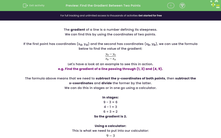# Find the Gradient Between Two Points

In this worksheet, students will find the gradients of straight lines when given the coordinates of two points on the line.Key stage:  KS 4

Year:  GCSE

GCSE Subjects:   Maths

GCSE Boards:   Pearson Edexcel, OCR, Eduqas, AQA,

Curriculum topic:   Algebra, Graphs of Equations and Functions

Curriculum subtopic:   Graphs Straight Line Graphs

Difficulty level:#### Worksheet Overview

The gradient of a line is a number defining its steepness.

We can find this by using the coordinates of two points.

If the first point has coordinates (xa, ya) and the second has coordinates (xb, yb), we can use the formula below to find the value of the gradient:Let's have a look at an example to see this in action.

e.g. Find the gradient of a line passing through (1, 3) and (4, 9).

The formula above means that we need to subtract the y-coordinates of both points, then subtract the x-coordinates and divide the former by the latter.

We can do this in stages or in one go using a calculator.

In stages:

9 - 3 = 6

4 - 1 = 3

6 ÷ 3 = 2

Using a calculator:

This is what we need to put into our calculator:In this activity, we will find the gradients of straight lines when given the coordinates of two points on the line.

If you prefer the second method in the options above, you may want to have a calculator handy to support you.

Want a bit more help with this before you begin? Why not watch this short video?

### What is EdPlace?

We're your National Curriculum aligned online education content provider helping each child succeed in English, maths and science from year 1 to GCSE. With an EdPlace account you’ll be able to track and measure progress, helping each child achieve their best. We build confidence and attainment by personalising each child’s learning at a level that suits them.

Get started